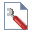Join one of our upcoming courses taking place around the world! Explore now

# Sensitivity Analysis

The effect of uncertainty or variability in the values of input parameters can be explored using a Sensitivity Analysis. In sensitivity analysis, model parameters selected by the user, are varied across a range of values and the effect on computed factors of safety observed. This helps to identify the parameters that have the most effect on the stability of a slope.

A Sensitivity Analysis in Slide2 works as follows:

1. To carry out a Sensitivity Analysis with Slide2, you must first select the Sensitivity Analysis checkbox on the Statistics page in the Project Settingsdialog.
2. A Statistics menu will then be available in the Slide2 main menu.
3. Use the options in the Statistics menu to select the input data variables that you would like to use in the Sensitivity Analysis, and specify a MINIMUM and MAXIMUM value for each variable you have selected.
4. In the input data dialogs, the minimum and maximum values are specified as RELATIVE distances from the mean value, because this simplifies the data input. During the analysis, these are converted to the actual minimum and maximum values, as follows:

MINIMUM = MEAN – Relative MINIMUM

MAXIMUM = MEAN + Relative MAXIMUM

EXAMPLE: if the Mean Friction Angle = 35, and the Relative Minimum = Relative Maximum = 10, then the actual Minimum = 25 degrees, and the actual Maximum = 45 degrees. These are the minimum and maximum values which are used in the analysis.

When you select Compute, the Sensitivity Analysis will be performed as follows:

5. For EACH variable that you have selected for the Sensitivity Analysis, the range between the MINIMUM and MAXIMUM values you have defined for the variable, will be divided into 50 equal intervals.
6. The safety factor for the Global Minimum slip surface, will be re-computed, for each of the 50 values of the variable, between the MINIMUM and MAXIMUM.
7. This process is repeated for EACH variable that you have selected for the Sensitivity Analysis.
8. When the Sensitivity Analysis is being performed on a variable, ALL OTHER VARIABLES are held constant, at their mean (deterministic) value. The Sensitivity analysis is only performed on one variable at a time, even if multiple variables have been selected for the analysis.
9. This results in a graph of safety factor, versus the variable between the MINIMUM and MAXIMUM values you have specified for the variable.
10. The Sensitivity Analysis plot is viewed in the Slide2 Interpret program. If you have selected more than one variable for the Sensitivity Analysis, ALL graphs for ALL variables will be plotted on the same graph. See the Sensitivity Plot topic for more information.

## Sensitivity Analysis vs. Probabilistic Analysis

It is important to note the following distinctions, and common features, between a Sensitivity Analysis and a Probabilistic Analysis with Slide2:

1. Although the Sensitivity Analysis option in Slide2 has been grouped with the Probabilistic Analysis option in the Project Settings dialog, a Sensitivity Analysis should NOT be confused with a Probabilistic Analysis.
2. A Probabilistic Analysis involves the statistical sampling of distributions that you have defined for your random variables. A Sensitivity Analysis simply involves the variation of a variable between minimum and maximum values.
3. A Sensitivity Analysis is performed on ONLY ONE VARIABLE AT A TIME. A Probabilistic Analysis uses sampled values of ALL random variables, for each iteration of the Probabilistic Analysis.
4. The method of selecting and defining variables for a Sensitivity Analysis, is the same as that for a Probabilistic Analysis. In both cases, the options in the Statistics menu are used.
5. The input data variables that you may select for a Sensitivity Analysis, are the same as those which you may use for a Probabilistic Analysis.
6. For a Sensitivity Analysis, it is only necessary to specify a MINIMUM and MAXIMUM value for each variable. For a Probabilistic Analysis, a standard deviation and statistical distribution are also selected (these are not applicable for a Sensitivity Analysis).
7. You may run BOTH a Sensitivity Analysis and a Probabilistic Analysis, at the same time, by selecting the checkbox for each option, in the Project Settings dialog.
8. Remember that the primary objective of a Probabilistic Analysis, is to calculate the Probability of Failure (or Reliability Index) for the slope. The primary objective of a Sensitivity Analysis, is to determine the effect of INDIVIDUAL VARIABLES on the safety factor.

## Data Input for Sensitivity Analysis

The general procedure for selecting variables and defining MINIMUM and MAXIMUM values, for a Sensitivity Analysis, is the same as the procedure used for selecting and defining the Random Variables for a Probabilistic Analysis. For details about these procedures, see the topics in the Probabilistic Analysis section of this Help system. NOTE the following:

1. If you are performing BOTH a Probabilistic Analysis AND a Sensitivity Analysis, then the Sensitivity Analysis will be performed, using the same variables that you have selected for the Probabilistic Analysis. The MINIMUM and MAXIMUM values you have defined for each random variable, will be used as the MINIMUM and MAXIMUM values for the Sensitivity Analysis.
2. Therefore, if you are performing BOTH a Probabilistic Analysis AND a Sensitivity Analysis, the Sensitivity Analysis simply uses the information you have specified for your Probabilistic Analysis, in order to perform the Sensitivity Analysis on the same variables.
3. If you are ONLY performing a Sensitivity Analysis, then the procedure for selecting and defining variables, is the same as described for the Probabilistic Analysis, except that standard deviations and statistical distributions are NOT specified, since they are NOT applicable, if you are ONLY running a Sensitivity Analysis.